This book is archived and will be removed July 6, 2022. Please use the updated version.

Nuclear Physics

# 73 Properties of Nuclei

### Learning Objectives

By the end of this section, you will be able to:

• Describe the composition and size of an atomic nucleus
• Use a nuclear symbol to express the composition of an atomic nucleus
• Explain why the number of neutrons is greater than protons in heavy nuclei
• Calculate the atomic mass of an element given its isotopes

The atomic nucleus is composed of protons and neutrons ((Figure)). Protons and neutrons have approximately the same mass, but protons carry one unit of positive charge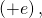and neutrons carry no charge. These particles are packed together into an extremely small space at the center of an atom. According to scattering experiments, the nucleus is spherical or ellipsoidal in shape, and about 1/100,000th the size of a hydrogen atom. If an atom were the size of a major league baseball stadium, the nucleus would be roughly the size of the baseball. Protons and neutrons within the nucleus are called nucleons.

The atomic nucleus is composed of protons and neutrons. Protons are shown in blue, and neutrons are shown in red.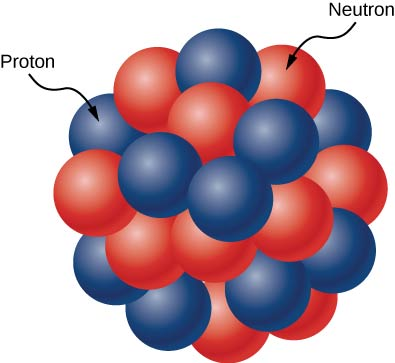### Counts of Nucleons

The number of protons in the nucleus is given by the atomic number, Z. The number of neutrons in the nucleus is the neutron number, N. The total number of nucleons is the mass number, A. These numbers are related by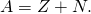A nucleus is represented symbolically by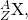where X represents the chemical element, A is the mass number, and Z is the atomic number. For example,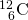represents the carbon nucleus with six protons and six neutrons (or 12 nucleons).

A graph of the number N of neutrons versus the number Z of protons for a range of stable nuclei (nuclides) is shown in (Figure). For a given value of Z, multiple values of N (blue points) are possible. For small values of Z, the number of neutrons equals the number of protons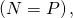and the data fall on the red line. For large values of Z, the number of neutrons is greater than the number of protons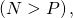and the data points fall above the red line. The number of neutrons is generally greater than the number of protons for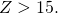This graph plots the number of neutrons N against the number of protons Z for stable atomic nuclei. Larger nuclei, have more neutrons than protons.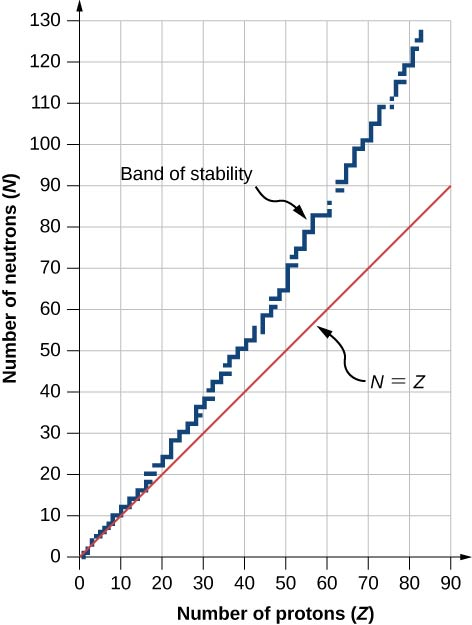A chart based on this graph that provides more detailed information about each nucleus is given in (Figure). This chart is called a chart of the nuclides. Each cell or tile represents a separate nucleus. The nuclei are arranged in order of ascending Z (along the horizontal direction) and ascending N (along the vertical direction).

Partial chart of the nuclides. For stable nuclei (dark blue backgrounds), cell values represent the percentage of nuclei found on Earth with the same atomic number (percent abundance). For the unstable nuclei, the number represents the half-life.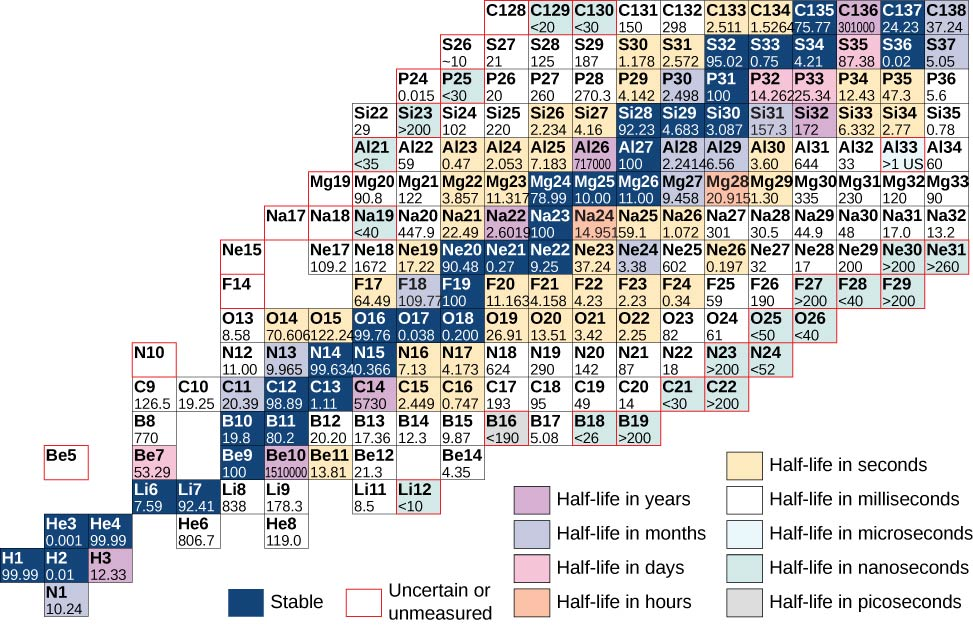Atoms that contain nuclei with the same number of protons (Z) and different numbers of neutrons (N) are called isotopes. For example, hydrogen has three isotopes: normal hydrogen (1 proton, no neutrons), deuterium (one proton and one neutron), and tritium (one proton and two neutrons). Isotopes of a given atom share the same chemical properties, since these properties are determined by interactions between the outer electrons of the atom, and not the nucleons. For example, water that contains deuterium rather than hydrogen (“heavy water”) looks and tastes like normal water. The following table shows a list of common isotopes.

Common Isotopes*No entry if less than 0.001 (trace amount).
**Stable if half-life > 10 seconds.
Element Symbol Mass Number Mass (Atomic Mass Units) Percent Abundance* Half-life**
Hydrogen H 1 1.0078 99.99 stable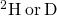2 2.0141 0.01 stable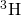3 3.0160 12.32 y
Carbon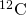12 12.0000 98.91 stable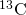13 13.0034 1.1 stable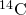14 14.0032 5730 y
Nitrogen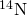14 14.0031 99.6 stable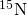15 15.0001 0.4 stable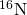16 16.0061 7.13 s
Oxygen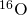16 15.9949 99.76 stable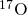17 16.9991 0.04 stable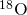18 17.9992 0.20 stable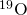19 19.0035 26.46 s

Why do neutrons outnumber protons in heavier nuclei ((Figure))? The answer to this question requires an understanding of forces inside the nucleus. Two types of forces exist: (1) the long-range electrostatic (Coulomb) force that makes the positively charged protons repel one another; and (2) the short-range strong nuclear force that makes all nucleons in the nucleus attract one another. You may also have heard of a “weak” nuclear force. This force is responsible for some nuclear decays, but as the name implies, it does not play a role in stabilizing the nucleus against the strong Coulomb repulsion it experiences. We discuss strong nuclear force in more detail in the next chapter when we cover particle physics. Nuclear stability occurs when the attractive forces between nucleons compensate for the repulsive, long-range electrostatic forces between all protons in the nucleus. For heavy nuclei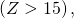excess neutrons are necessary to keep the electrostatic interactions from breaking the nucleus apart, as shown in (Figure).

(a) The electrostatic force is repulsive and has long range. The arrows represent outward forces on protons (in blue) at the nuclear surface by a proton (also in blue) at the center. (b) The strong nuclear force acts between neighboring nucleons. The arrows represent attractive forces exerted by a neutron (in red) on its nearest neighbors.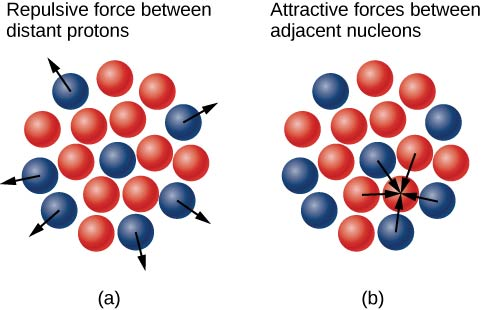Because of the existence of stable isotopes, we must take special care when quoting the mass of an element. For example, Copper (Cu) has two stable isotopes: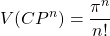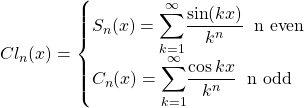Given these two “versions” of Cu, what is the mass of this element? The atomic mass of an element is defined as the weighted average of the masses of its isotopes. Thus, the atomic mass of Cu is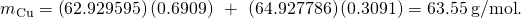The mass of an individual nucleus is often expressed in atomic mass units (u), where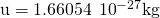. (An atomic mass unit is defined as 1/12th the mass of anucleus.) In atomic mass units, the mass of a helium nucleus (A = 4) is approximately 4 u. A helium nucleus is also called an alpha (α) particle.

### Nuclear Size

The simplest model of the nucleus is a densely packed sphere of nucleons. The volume V of the nucleus is therefore proportional to the number of nucleons A, expressed by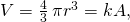where r is the radius of a nucleus and k is a constant with units of volume. Solving for r, we have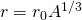where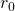is a constant. For hydrogen (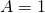),corresponds to the radius of a single proton. Scattering experiments support this general relationship for a wide range of nuclei, and they imply that neutrons have approximately the same radius as protons. The experimentally measured value foris approximately 1.2 femtometer (recall that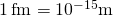).

The Iron NucleusFind the radius (r) and approximate density (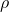) of a Fe-56 nucleus. Assume the mass of the Fe-56 nucleus is approximately 56 u.

Strategy (a) Finding the radius of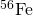is a straightforward application of, given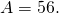(b) To find the approximate density of this nucleus, assume the nucleus is spherical. Calculate its volume using the radius found in part (a), and then find its density from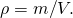Solution

1. The radius of a nucleus is given by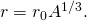Substituting the values forand A yields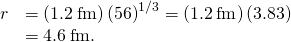2. Density is defined to be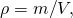which for a sphere of radius r isSubstituting known values gives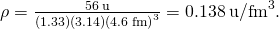Converting to units of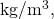we find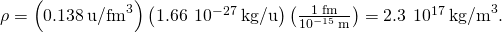Significance

1. The radius of the Fe-56 nucleus is found to be approximately 5 fm, so its diameter is about 10 fm, or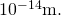In previous discussions of Rutherford’s scattering experiments, a light nucleus was estimated to be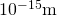in diameter. Therefore, the result shown for a mid-sized nucleus is reasonable.
2. The density found here may seem incredible. However, it is consistent with earlier comments about the nucleus containing nearly all of the mass of the atom in a tiny region of space. One cubic meter of nuclear matter has the same mass as a cube of water 61 km on each side.

Check Your Understanding Nucleus X is two times larger than nucleus Y. What is the ratio of their atomic masses?

eight

### Summary

• The atomic nucleus is composed of protons and neutrons.
• The number of protons in the nucleus is given by the atomic number, Z. The number of neutrons in the nucleus is the neutron number, N. The number of nucleons is mass number, A.
• Atomic nuclei with the same atomic number, Z, but different neutron numbers, N, are isotopes of the same element.
• The atomic mass of an element is the weighted average of the masses of its isotopes.

### Conceptual Questions

Define and make clear distinctions between the terms neutron, nucleon, nucleus, and nuclide.

The nucleus of an atom is made of one or more nucleons. A nucleon refers to either a proton or neutron. A nuclide is a stable nucleus.

What are isotopes? Why do isotopes of the same atom share the same chemical properties?

### Problems

Find the atomic numbers, mass numbers, and neutron numbers for (a)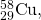(b)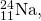(c)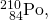(d)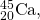and (e)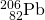.

Use the ruleAtomic Number (Z) Neutron Number (N) Mass Number (A)
(a) 29 29 58
(b) 11 13 24
(c) 84 126 210
(d) 20 25 45
(e) 82 124 206

Silver has two stable isotopes. The nucleus,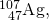has atomic mass 106.905095 g/mol with an abundance of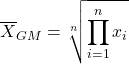; whereas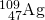has atomic mass 108.904754 g/mol with an abundance of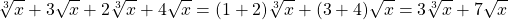. Find the atomic mass of the element silver.

The mass (M) and the radius (r) of a nucleus can be expressed in terms of the mass number, A. (a) Show that the density of a nucleus is independent of A. (b) Calculate the density of a gold (Au) nucleus. Compare your answer to that for iron (Fe).

a.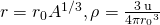;
b.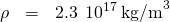A particle has a mass equal to 10 u. If this mass is converted completely into energy, how much energy is released? Express your answer in mega-electron volts (MeV). (Recall that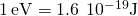.)

Find the length of a side of a cube having a mass of 1.0 kg and the density of nuclear matter.

side length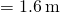The detail that you can observe using a probe is limited by its wavelength. Calculate the energy of a particle that has a wavelength of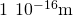, small enough to detect details about one-tenth the size of a nucleon.

### Glossary

atomic mass
total mass of the protons, neutrons, and electrons in a single atom
atomic mass unit
unit used to express the mass of an individual nucleus, where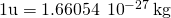atomic nucleus
tightly packed group of nucleons at the center of an atom
atomic number
number of protons in a nucleus
chart of the nuclides
graph comprising stable and unstable nuclei
isotopes
nuclei having the same number of protons but different numbers of neutrons
mass number
number of nucleons in a nucleus
neutron number
number of neutrons in a nucleus
nucleons
protons and neutrons found inside the nucleus of an atom
nuclide
nucleus
radius of a nucleus is defined as